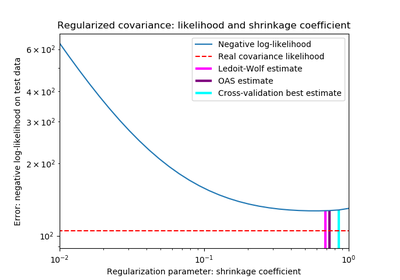# `sklearn.covariance`.empirical_covariance¶

`sklearn.covariance.``empirical_covariance`(X, assume_centered=False)[source]

Computes the Maximum likelihood covariance estimator

Parameters: X : ndarray, shape (n_samples, n_features) Data from which to compute the covariance estimate assume_centered : Boolean If True, data are not centered before computation. Useful when working with data whose mean is almost, but not exactly zero. If False, data are centered before computation. covariance : 2D ndarray, shape (n_features, n_features) Empirical covariance (Maximum Likelihood Estimator).

## Examples using `sklearn.covariance.empirical_covariance`¶Shrinkage covariance estimation: LedoitWolf vs OAS and max-likelihood